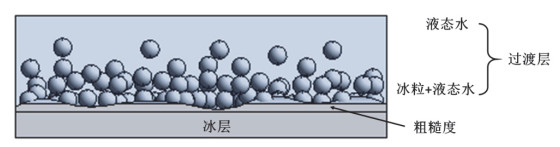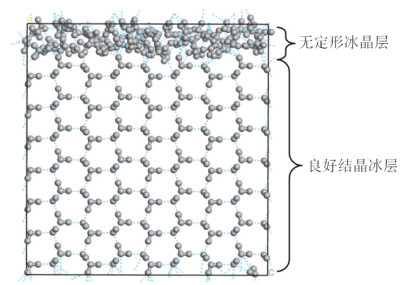## Reasons for the Low Frictional Coefficient of Ice Surface: Progress and Model Analysis

, , ,,Abstract

Low friction is the most outstanding feature of ice surface. The existence of liquid water on ice surface, which serves as lubricant, has been taken as the main cause for its low friction. Three main hypotheses for the formation of liquid water on ice surface were introduced and analyzed. It was found that the pressure-melting, friction heat-melting and pre-melting theories cannot give satisfactory explanation to all facts. Based on the previous studies, it was clear that the formation of liquid water was not the only reason for explaining the low friction of ice. A new model that there is a transition layer with small ice particles and liquid water forming a gel-like amorphous structure on the ice surface is proposed. Owing to the weak interaction between these small ice particles, they can easily slide or rotate along the shear direction and thus reduce friction. Both the rotation of ice particles and lubrication of liquid water benefit excellent slipperiness of ice surface.

Keywords： Ice surface ; Low frictional efficient ; Surface structure of ice ; Transition layer

WANG Guangzhen, WANG Wenliang, GAO Xin, ZHANG Shuyong. Reasons for the Low Frictional Coefficient of Ice Surface: Progress and Model Analysis. University Chemistry[J], 2019, 34(1): 33-38 doi:10.3866/PKU.DXHX201805022

## 1 引言

### 2.1 压力融解说

1850年Thomson发现了熔点和压力的线性关系。1886年Joly将该原理应用于解释冰的润滑现象，建立了“压力融解说”(pressure-melting theory)。Joly的计算表明，当冰面压力达到4.72 × 107 Pa时，冰的熔点可降低到-3.5 ℃ 。如果此时气温高于-3.5 ℃，则冰会在冰刀的压力下融化为水并起到润滑作用。当滑冰者离开这一区域时，融化的水会重新凝结成冰。“压力融解说”曾经在很长时间里作为压力对熔点影响的案例出现在国内外的物理化学教材中，如一本教学参考资料取冰刀的长度为7.62 cm，宽度为0.0245 mm，由此计算的压力为1.575 × 108 Pa，得出冰的熔点会相应降低10.95 K的结论

$\Delta p = \frac{{\Delta {H_{\rm{m}}}}}{{\Delta {V_{\rm{m}}}}}\ln \frac{T}{{{T_1}}}$

$\begin{array}{c}\Delta {V_{\rm{m}}} = {V_{\rm{m}}}({\rm{l}})-{V_{\rm{m}}}({\rm{s}}) = 0.018{\rm{ kg}} \cdot {\rm{mo}}{{\rm{l}}^{-{\rm{1}}}}(\frac{1}{{1000{\rm{ kg}} \cdot {{\rm{m}}^{-{\rm{3}}}}}} - \frac{1}{{920{\rm{ kg}} \cdot {{\rm{m}}^{ - 3}}}})\\ = - 1.565 \times {10^{ - 6}}{\rm{ }}{{\rm{m}}^{\rm{3}}} \cdot {\rm{mo}}{{\rm{l}}^{ - {\rm{1}}}}\end{array}$

### 2.2 摩擦融解说

“摩擦融解说”最早是由Bowden和Hughes于1939年提出的。他们通过计算证明冰刀产生的压力不足以使冰在低温下融解，“压力融解效应”只在接近冰的熔点时才存在。AngelPeña也将滑冰选手在冰面上滑行时冰刀与冰面快速摩擦所产生的热量作为冰熔融的主要原因。之后Colbeck [7-9]证明当冰刀划过冰面时，冰刀的温度会升高，且随着滑行速度的增加，温度升高更加显著。这与“压力融解”导致冰融化时伴随温度降低相抵触，进一步支持了“摩擦融解”的观点。

$f = \mu F = 0.02 \times 60{\rm{ kg}} \times 9.81{\rm{ m}} \cdot {{\rm{s}}^{-2}} = 11.772{\rm{ N}}$

${W_{\rm{f}}} = f \times l = 11.772{\rm{ N}} \times {\rm{1 m}} = 11.772{\rm{ J}}$

### 图1### 图2### 图3## 参考文献 原文顺序 文献年度倒序 文中引用次数倒序 被引期刊影响因子

Amit G. New Scientist 2015, 227 (3037), 38.

Rosenberg R. Physics Today 2005, 58 (12), 50.

Bowden F. P. ; Hughes T. P. Proc. Roy. Soc. Lond. A 1939, 172 (949), 280.

Colbeck S. C. ; Richtermenge J. A. ; Colveck S. C. ; Jezek K. C. Rev. Geophys. 1991, 29 (51), 218.

Colbeck S. C. Am. J. Phys. 1995, 63 (10), 888.

Colbeck S. C. ; Najarian L. ; Smith H. B. Am. J. Phys. 1997, 65 (6), 488.

Persson B. N. J. J. Chem. Phys. 2015, 143 (22), 1.

Thomson J. Proc. R. Soc. Lond. 1859, 10, 151.

Faraday M. Philos. Mag. Ser. 1859, 4 (17), 162.

Faraday M. Proc. R. Soc. 1860, 10, 440.

Gurney C. Proc. Phys. Soc. Ser. A 1949, 62 (358), 639.

Weyl W. A. J. Colloid Sci. 1951, 6 (5), 389.

Hosler C. L. ; Hallgren R. E. Discuss. Faraday Soc. 1961, 30, 200.

Orem M.W. J. Colloid Interface Sci. 1969, 31 (2), 278.

Kvlividze V. I. ; Kiselev V. F. ; Kurzaev A. B. ; Ushakova L. A. Surf. Sci. 1974, 44 (1), 60.

van Leeuwen J. M. J. Scipost Phys. 2017, 3 (6), 1.

Edward L. ; Krzysztof S. ; Louis P. J. Offshore and Polar Engin. 2014, 24 (1), 52.

Persson B. N. J. J. Chem. Phys. 2015, 143 (22), 224701.

Oxtoby D. W. Nature 1990, 347 (6295), 725.

/

 〈〉